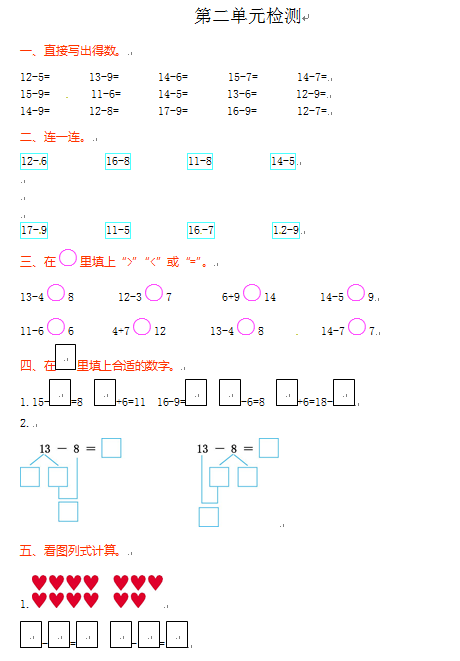12-5=　　　 13-9=　　　 14-6=　　 　15-7=　　　 14-7=

15-9=           11-6=　　      14-5=　　       13-6=           12-9=

14-9=　　       12-8=           17-9=　　       16-9=　　       12-7=

【DOC文档4页】 小学人教新课标数学一年级下学期第2单元测试卷2含答案A4电子版资料_可直接打印_会员免费下载##### 评论信息